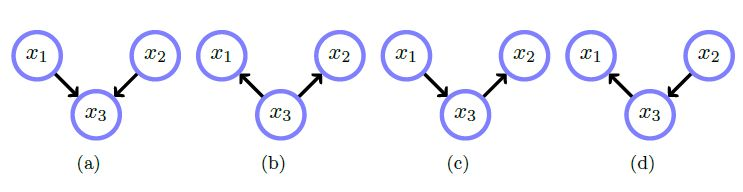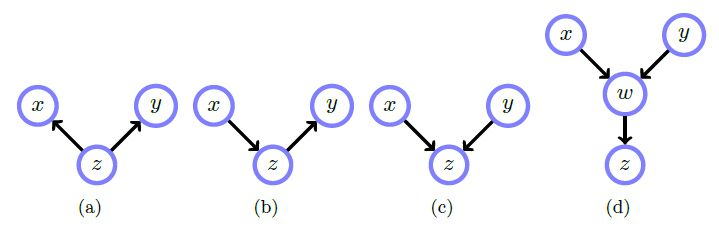Math and science::INF ML AI

# Belief networks: independence

It's not immediately obvious how to interpret the conditional relationships represented by a belief network. For example, consider the networks below.Network(s) [...] represent the distribution:

\begin{aligned}p(x_1, x_2, x_3) &= p(x_1 \vert x_3)p(x_2 \vert x_3)p(x_3) \\&= p(x_2 \vert x_3)p(x_3 \vert x_1)p(x_1) \\&= p(x_1 \vert x_3)p(x_3 \vert x_2)p(x_2) \end{aligned}

Network(s) [...] represent the distribution:

$p(x_1, x_2, x_3) = p(x_3 \vert x_1, x_2)p(x_1)p(x_2)$

Next, consider conditional independence.• In a), $$x$$ and $$y$$ are unconditionally dependent, and conditioned on $$z$$ they are independent. Knowing either gives information on the distribution of $$z$$, which in turn informs of the others distribution.. $$p(x, y \vert z) = p(x \vert z)p(y \vert z)$$
• In b), $$x$$ and $$y$$ are unconditionally dependent, and conditioned on $$z$$ they are independent. $$p(x, y \vert z) \varpropto p(z \vert x)p(x)p(y \vert z)$$
• In c), $$x$$ and $$y$$ are unconditionally independent, and conditioned on $$z$$ they are dependent. $$p(x, y \vert z) \varpropto p(z \vert x, y)p(x)p(y)$$
• Id d), $$x$$ and $$y$$ are unconditionally independent, and conditioned on $$z$$ or $$w$$, are dependent. See book for full equation.